Next: Electrostatics in Dielectric Media Up: Potential Theory Previous: Poisson's Equation in Cylindrical

# Exercises

1. Two concentric spheres have radii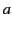,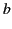(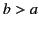) and are each divided into two hemispheres by the same horizontal plane. The upper hemisphere of the inner sphere and the lower hemisphere of the outer sphere are maintained at potential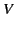. The other hemispheres are at zero potential. Demonstrate that the potential in the region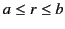can be written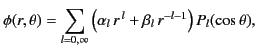where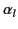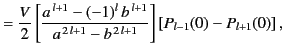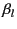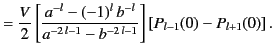Here,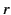,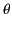,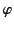are conventional spherical coordinates whose origin coincides with the common center of the spheres, and are such that the dividing plane corresponds to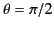.

2. A spherical surface of radius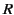has charge uniformly distributed over its surface with density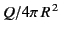, except for a spherical cap at the north pole, defined by the cone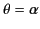. Here,,,are conventional spherical coordinates whose origin coincides with the center of the surface.
1. Show that the potential inside the spherical surface can be expressed as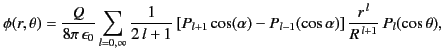where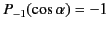.
2. Show that the electric field at the origin is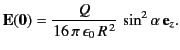3. Show that in the limit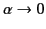,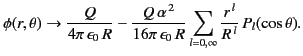4. Show that in the limit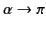,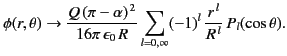3. The Dirichlet Green's function for the unbounded space between planes at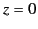and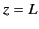allows a discussion of a point charge, or a distribution of charge, between parallel conducting planes held at zero potential.
1. Using cylindrical coordinates, show that one form of the Green's function is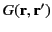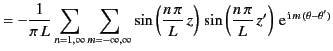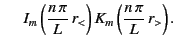2. Show that an alternative form of the Green's function is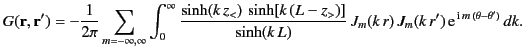4. From the results of the previous exercise, show that the potential due to a point charge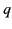placed between two infinite parallel conducting planes held at zero potential can be written as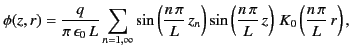where the planes are atand, and the charge is on the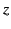-axis at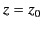. Show that induced surface charge densities on the lower and upper planes are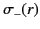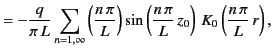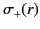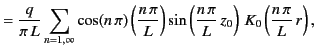respectively.

5. Show that the potential due to a conducting disk of radiuscarrying a chargeis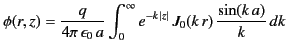in cylindrical coordinates (whose origin coincides with the center of the disk, and whose symmetry axis coincides with that of the disk.)

6. A conducting spherical shell of radiusis placed in a uniform electric field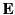. Show that the force tending to separate two halves of the sphere across a diametral plane perpendicular tois given by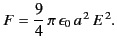Next: Electrostatics in Dielectric Media Up: Potential Theory Previous: Poisson's Equation in Cylindrical
Richard Fitzpatrick 2014-06-27